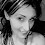--># Physicists Prove That 'Paradox-Free' Time Travel Is Mathematically Possible

Physicists have long studied the potential of time travel, which appears to be an impossible feat in the eyes of many people. While the practicality of such an event is still a long way off, recently, scientists have demonstrated that time travel is theoretically possible.

After solving a logical paradox, Australian scientists claim to have proved that time travel is theoretically possible.

Dr. Costa and Germain Tobar, a student at the University of Queensland's School of Mathematics and Physics, have proposed a mathematical model that proves paradox-free time travel is theoretically possible.

Dr. Costa and Germain Tobar used a mathematical model to reconcile Einstein's general theory of relativity with classical dynamics.

Essentially, there is an important paradox known as the grandfather paradox that exists between Einstein's famous theory of general relativity and the world of classical dynamics. This paradox is a well-known flaw in time travel caused by the clash of these two systems.

Without delving too deeply into those specific theories, the important thing to note is that Einstein's theory allows for the possibility of a person traveling back in time to kill their grandfather using a time loop before their grandfather had children. According to classical dynamics, the sequence of events following the grandfather's death would also make the traveler's own birth impossible.

“Classical dynamics says if you know the state of a system at a particular time, this can tell us the entire history of the system,” said Germain Tobar.

“This has a wide range of applications, from allowing us to send rockets to other planets and modeling how fluids flow.”

“For example, if I know the current position and velocity of an object falling under the force of gravity, I can calculate where it will be at any time.”

“However, Einstein’s theory of general relativity predicts the existence of time loops or time travel — where an event can be both in the past and future of itself — theoretically turning the study of dynamics on its head.”

Tobar said A unified theory that reconciles both traditional dynamics and Einstein's Theory of Relativity could be one possible solution.

"But the current science says both theories cannot both be true," he said.

Tobar says he has been perplexed for years about how "the science of dynamics can square with Einstein's predictions." "Is time travel mathematically possible?" he wondered.

Tobar and Costa claim to have found a way to "square the numbers" to determine whether such time travel is theoretically possible.

“The math checks out – and the results are science fiction,” Dr. Costa said.

Mr. Tobar and Dr. Costa used the coronavirus pandemic as a model to determine whether the two theories could coexist.

They envisioned a time traveler attempting to prevent patient zero from becoming infected with Covid-19.

Einstein's theory allows for time travel, but the science of dynamics dictates that the fundamental sequence of events cannot be disrupted.

This is because if the time traveler successfully prevented the virus from spreading, it would negate their initial motivation for traveling back in time.

“This is a paradox – an inconsistency that often leads people to think that time travel cannot occur in our universe,” Dr. Costa said.

“Some physicists say it is possible, but logically it’s hard to accept because that would affect our freedom to make any arbitrary action. It would mean you can time travel, but you cannot do anything that would cause a paradox to occur.”

“In the coronavirus patient zero example, you might try and stop patient zero from becoming infected, but in doing so you would catch the virus and become patient zero, or someone else would,” Tobar said.

“No matter what you did, the salient events would just recalibrate around you,” he added.

“This would mean that – no matter your actions - the pandemic would occur, giving your younger self the motivation to go back and stop it,” he said.

It would imply that you can time travel but cannot do anything that would result in a paradox.

According to their research, events will align themselves logically to eliminate any inconsistency in the universe, making time travel at free will possible. The research is published in Classical and Quantum Gravity.

### 2 Responses to "Physicists Prove That 'Paradox-Free' Time Travel Is Mathematically Possible"

1.Law of gravity-two bodies attracted each other in direction proportion to their masses inversely to the square of the distance between them.
Formula:F=GMm/r², where G=6.67×10-¹¹Nm²/kg², M-Mass(larger)object,m-mass of 2nd object (smaller).r=Radial distance between M.___center___.m, GMm=Units kg, F=Force

2.Quantum Mechanics Time Travel:
Center of gravity d=WD/W+W(3.00×10(8)Ms=186.000mps
Centrifugal Force d=wD/w+W
E=Mass centri², F=.000034×3×2×5∞(infinit)=510lbs.ans
Energy(E)=WV^/2g, G=32.16 V=32.16
E=the constant light transmitted to Earth 5.52 kg/dm3, E=50×100^/2×32.16=7.774ft_lbs.ans
F=.000034Wrn^, M=mass of Earth;AI mean, molecular weight of A.I.
E=Mc^ 4th 200.200 ,🎗️=Mc^ velocity of flow(Ea)=active energy E=.707;volt=10% EMax=110/.707🎗️